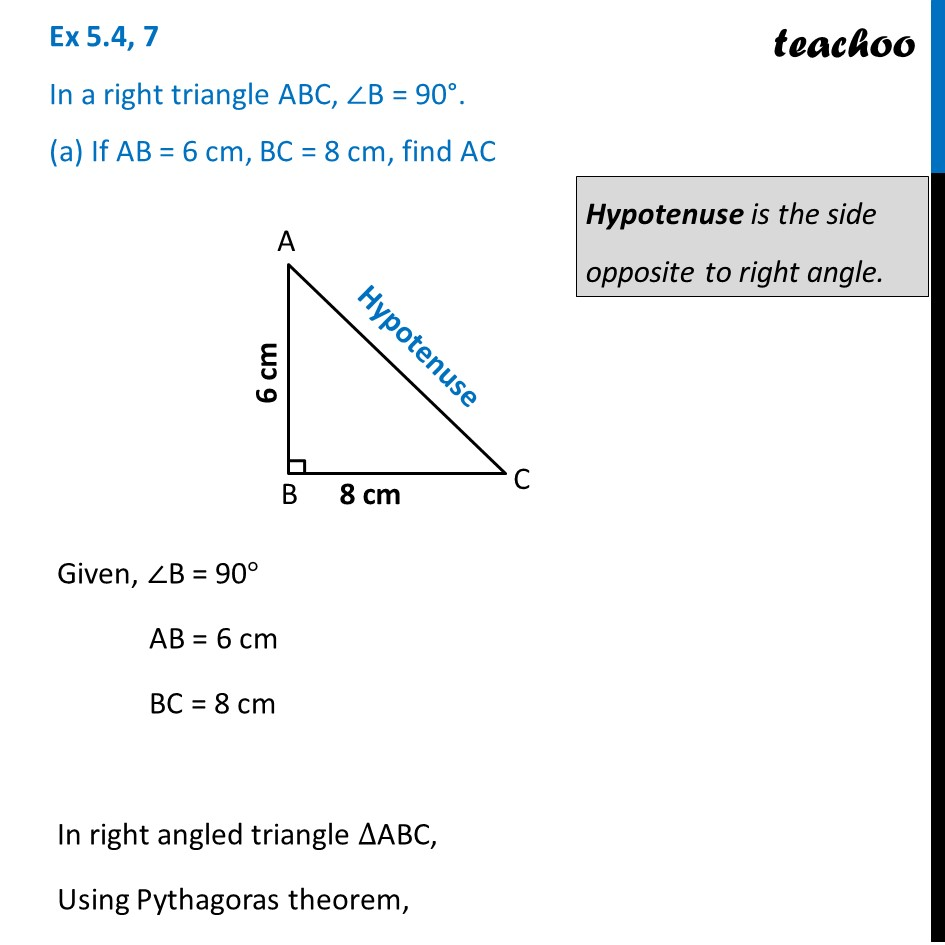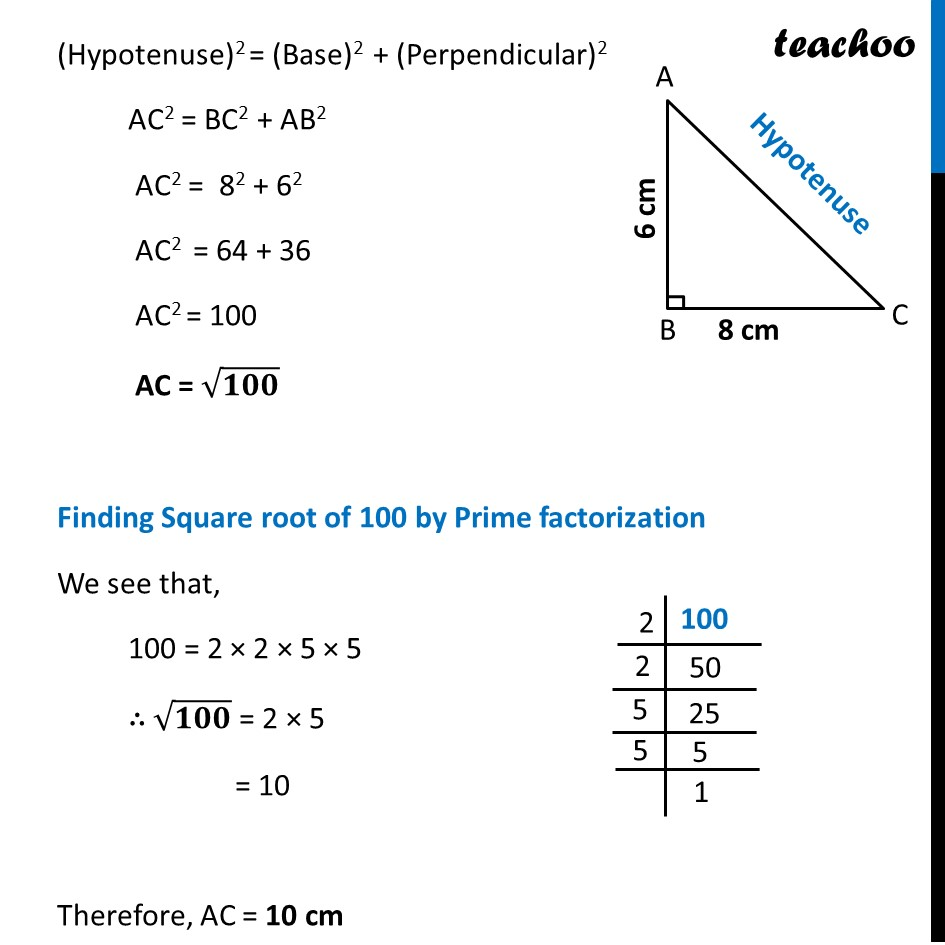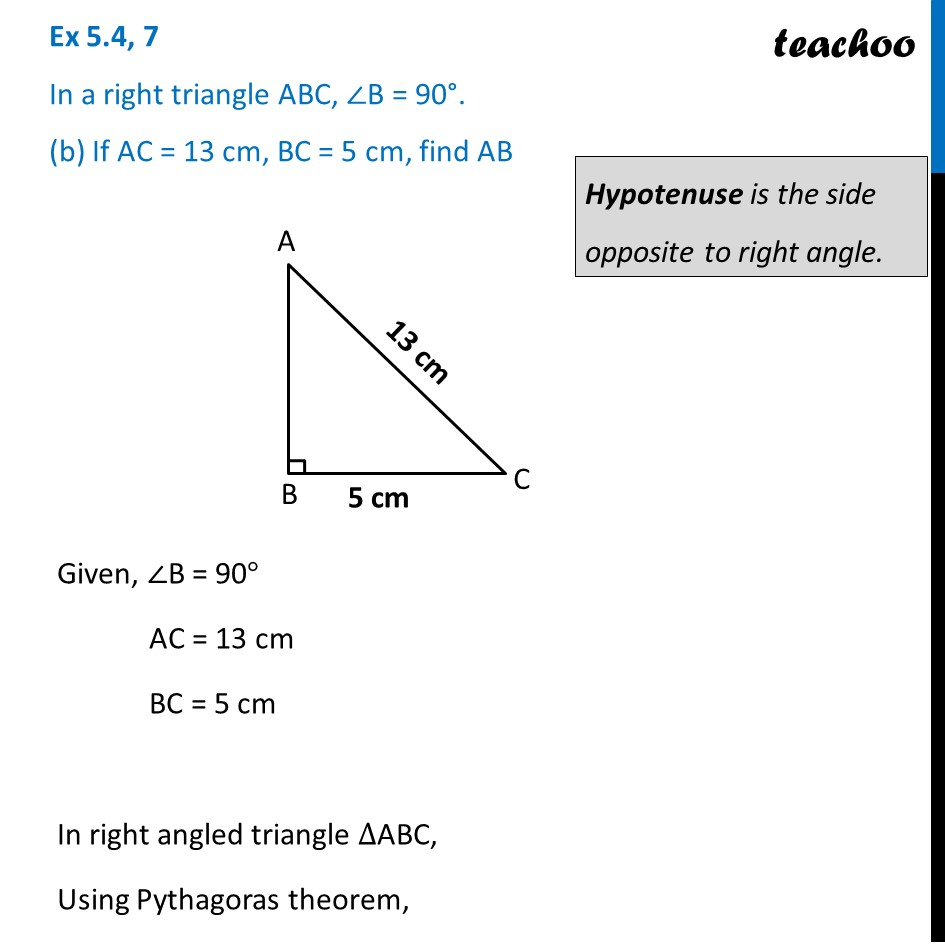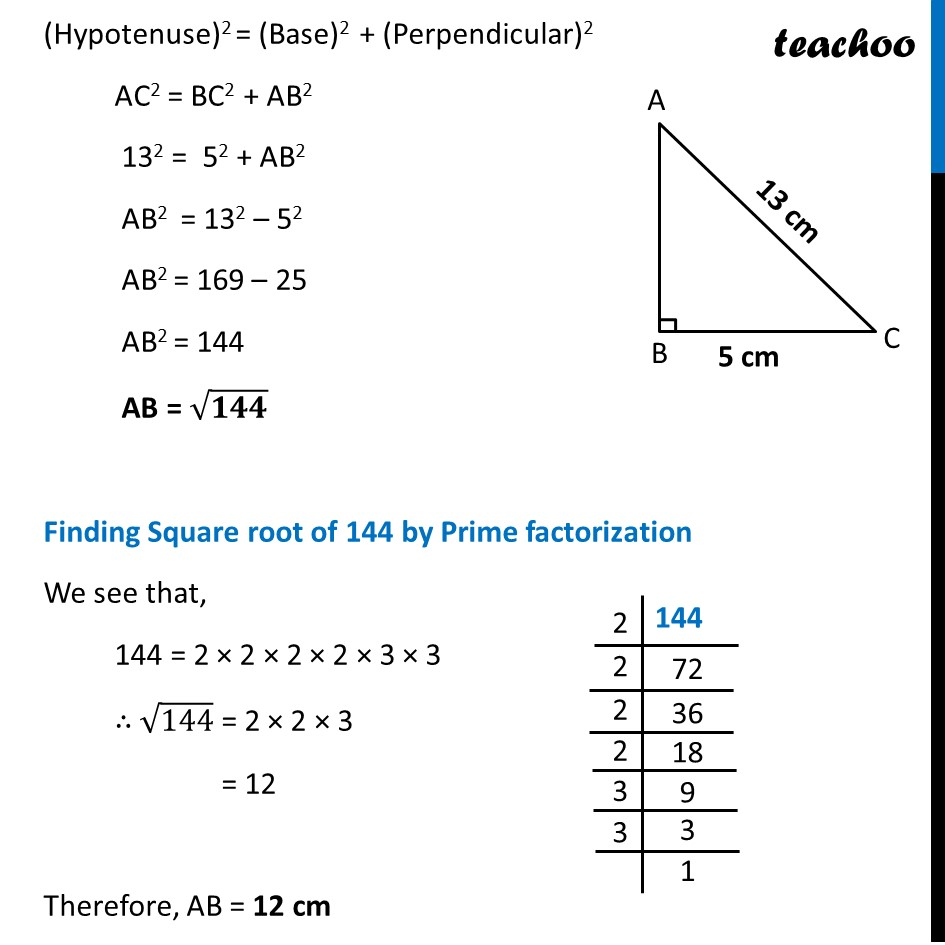Statement Questions

Chapter 5 Class 8 Squares and Square Roots
Concept wiseLearn in your speed, with individual attention - Teachoo Maths 1-on-1 Class

### Transcript

Ex 5.4, 7 In a right triangle ABC, ∠B = 90°. (a) If AB = 6 cm, BC = 8 cm, find AC Given, ∠B = 90° AB = 6 cm BC = 8 cm In right angled triangle ∆ABC, Using Pythagoras theorem, Hypotenuse is the side opposite to right angle. (Hypotenuse)2 = (Base)2 + (Perpendicular)2 AC2 = BC2 + AB2 AC2 = 82 + 62 AC2 = 64 + 36 AC2 = 100 AC = √𝟏𝟎𝟎 Finding Square root of 100 by Prime factorization We see that, 100 = 2 × 2 × 5 × 5 ∴ √𝟏𝟎𝟎 = 2 × 5 = 10 Therefore, AC = 10 cm Ex 5.4, 7 In a right triangle ABC, ∠B = 90°. (b) If AC = 13 cm, BC = 5 cm, find AB Given, ∠B = 90° AC = 13 cm BC = 5 cm In right angled triangle ∆ABC, Using Pythagoras theorem, (Hypotenuse)2 = (Base)2 + (Perpendicular)2 AC2 = BC2 + AB2 132 = 52 + AB2 AB2 = 132 – 52 AB2 = 169 – 25 AB2 = 144 AB = √𝟏𝟒𝟒 Finding Square root of 144 by Prime factorization We see that, 144 = 2 × 2 × 2 × 2 × 3 × 3 ∴ √144 = 2 × 2 × 3 = 12 Therefore, AB = 12 cm